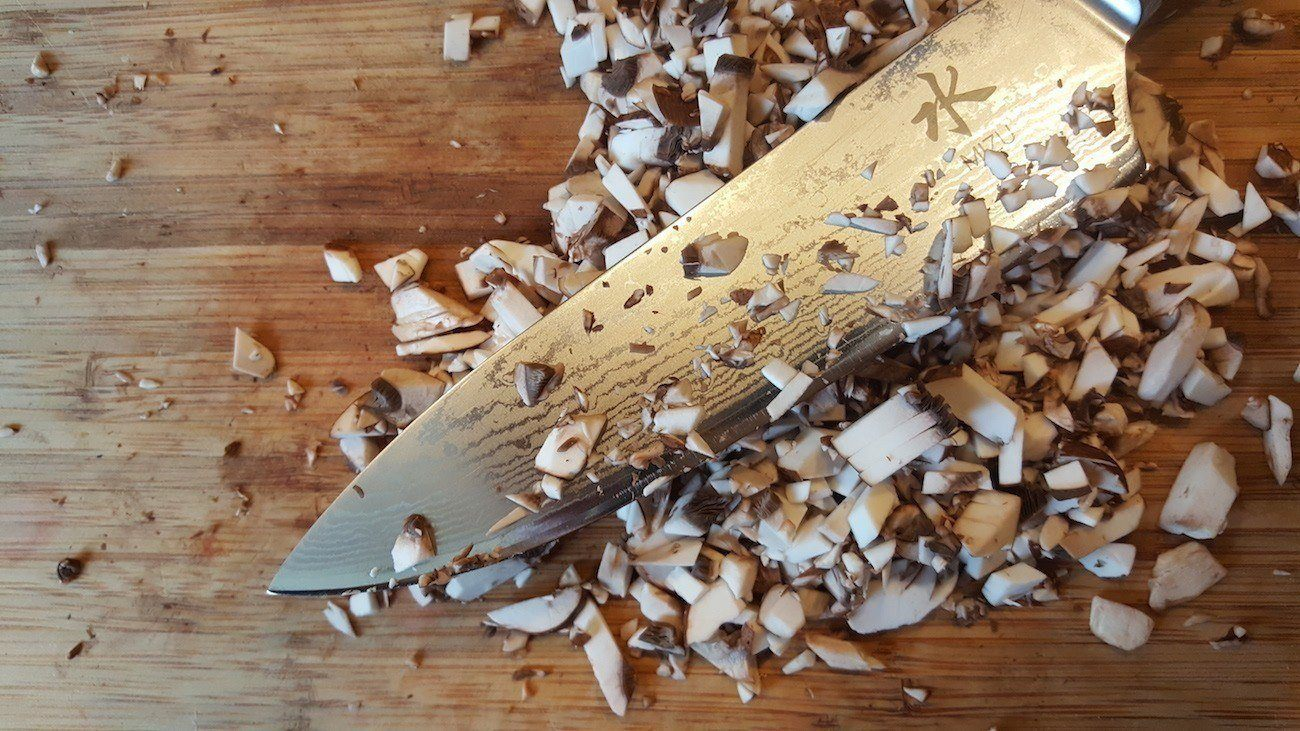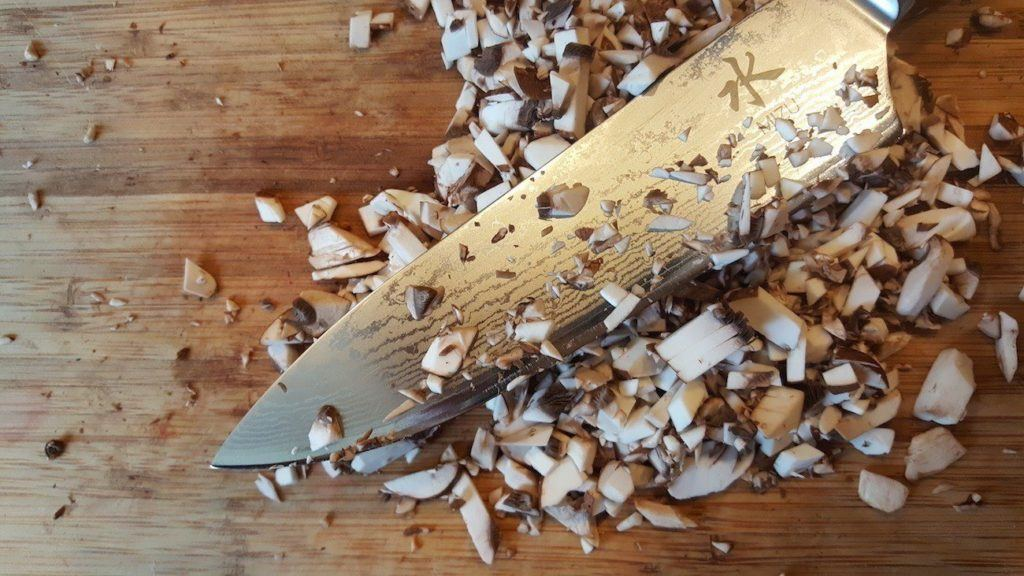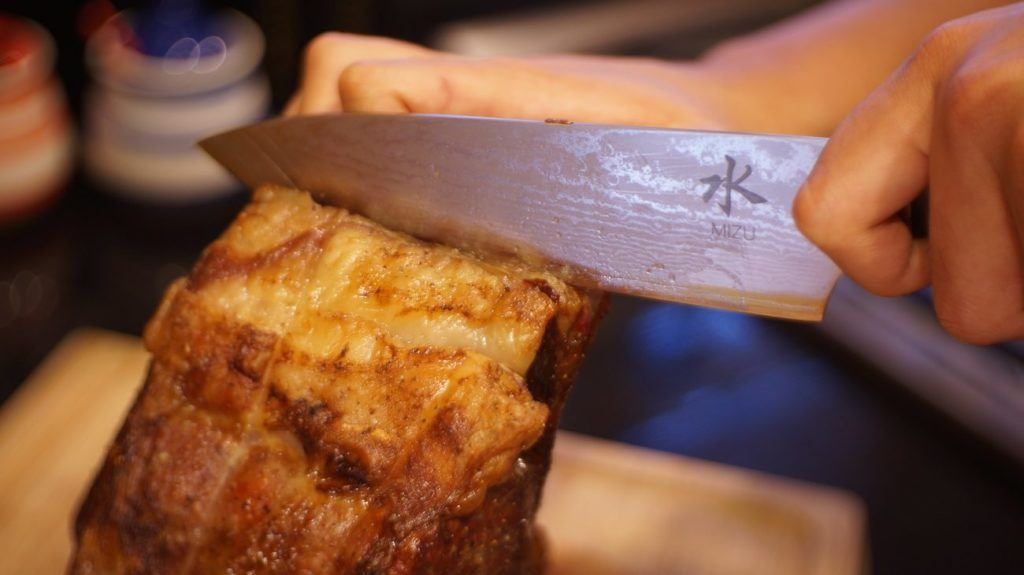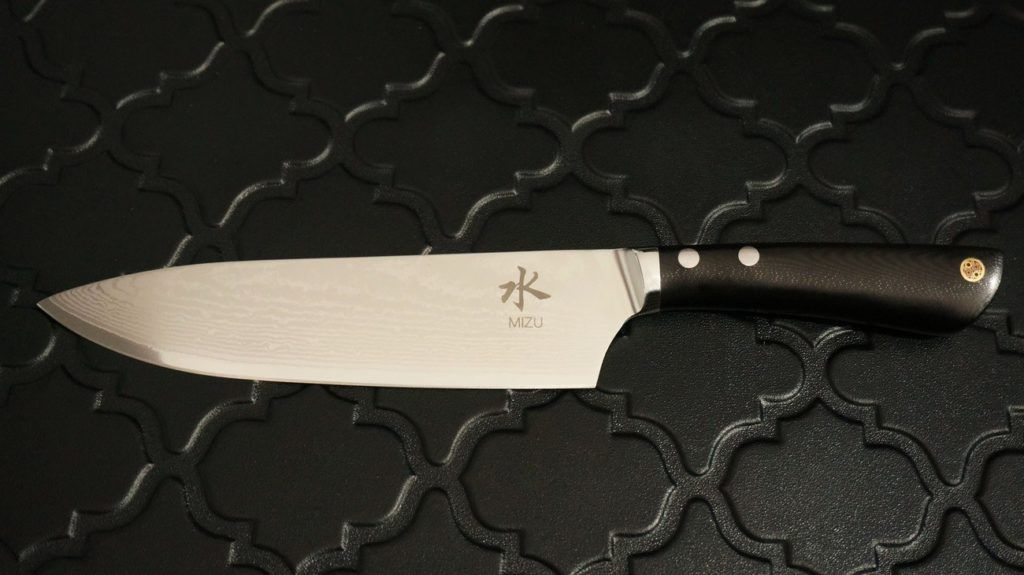The Mizu premium chef’s knife is affordable and a must-have in your kitchenThey say a great chef’s skill isn’t down to the appliances and tools but due to their passion and creativity. While a budget stove or decades-old pots and pans won’t affect the quality of your cooking, there is certainly one item every modern kitchen should have: a great knife. However, most on the market are alarmingly expensive. But, the Mizu premium Chef’s Knife:
– offers exceptional functionality
– is professional grade
– uses fine materials
– is totally affordable

The Mizu Premium Chef’s Knife

As you may know, a sharp knife is, in fact, much safer than a dull one. This is because a sharp knife allows for smooth and controlled cuts while a dull one does not. The Mizu Chef’s Knife achieves its extra sharp design by utilizing high carbon VG10 Damascus steel. This is a premium metal and gives you the ability to slice and dice like a real Fruit Ninja. In addition to exceptional cutting, the Mizu Chef’s Knife is easy to keep sharp. With the blade measuring a full 8 inches, this masterful knife is complete with a pointed tip and a hardness rating of 60-62. All of these features give you an effortlessly easy experience.High-Quality Materials

However, the Mizu Chef’s Knife isn’t just another sharp knife in your drawer. It features a G10 synthetic fiber resin handle that is both highly durable and ergonomic. Holding the blade in place is a slanted bolster as well as dual stainless steel rivets. In addition, the 15-degree dual bevel works for both right and left-handed users. No matter what you’re cutting, you get an ergonomic and well-balanced feel every time.Approved by Professionals

There is a multitude of features that set the Mizu Chef’s Knife a cut above the rest. But, it’s the approval by professional chefs that really seals the deal. The pros love the robust weight and are blown away at how easy it is to sharpen. They are all uniformly shocked by the price. Considering the material, quality, sharpness, and ease of use, it’s hard to believe the Mizu Chef’s Knife is an affordable household item. But, the creators cut the distributors to guarantee a low price.What We ❤️️

This knife ticks all the boxes. No matter your skill level, your cooking deserves the Mizu Chef’s Knife.

Future Designs

This knife is perfect in every way. We can only hope the company brings this sophistication to an entire range of knives

Availability

– Kickstarter: until May 15th
Pledge: \$65
– Delivery: September 2017

Meet Ashley Timms

Ashley Timms is the Editor in Chief at Gadget Flow where she gets to write about the most incredible products in the world. When she’s not writing, she’s producing fine art dog photography.

Click here to tag users that participate in this comment thread.
Click or drag your image here (Maximum Size 4MB, Accepted formats JPG, PNG, GIF).
• 😂
• ❤️
• ♥️
• 😍
• 😭
• 😊
• 😒
• 😘
• 💕
• ☺️
• 😩
• 👌
• 😔
• 😏
• 😁
• 😉
• 👍
• 🙏
• 😌
• 🎶
• 😳
• 🙌
• 🙈
• 😢
• 😎
• ✌️
• 👀
• 😅
• 😴
• 😄
• 💜
• 💔
• 💯
• 😑
• 💖
• 💙
• 😕
• 💁
• 😜
• 😞
• 😋
• 😐
• 😪
• 👏
• 💘
• 💗
• 💞
• ⬅️
• 🙊
• 💋
• 👉
• 🌸
• 😱
• 🔥
• 😡
• 😃
• 🎉
• 👊
• 😫
• 📷
• 🌹
• 😝
• 💪
• 💀
• ☀️
• 💛
• 😤
• 🌚
• 😆
• 😓
• 👈
• ✔️
• 😻
• 😀
• 😷
• 💚
• 👋
• 😣
• 💓
• ▶️
• ◀️
• ↪️
• ↩️
• 👑
• 😚
• 😛
• 😥
• 😇
• 🎧
• 😖
• 😠
• 😬
• 🌟
• 🔫
• 🙋
• 👎
• 💃
• 🎵
• 😶
• 💫
• 👇
• 🔴
• 🙅
• 💥
• 💭
• 👅
• 💩
• 😰
• 💎
• 🙆
• 🍕
• 😹
• 🌞
• 🍃
• 💦
• 🐧
• 💤
• 🚶
• ✈️
• 🎈
• 🎀
• ☑️
• 😟
• 🔞
• 😨
• 🍀
• 🌺
• 🎤
• 👐
• 👻
• 🌴
• ‼️
• 💅
• 👽
• 🙇
• 👼
• 👯
• ❄️
• ☝️
• 😙
• 🌈
• 🌙
• 💟
• 💝
• 🎁
• 🍻
• 😧
• 🌍
• 🎥
• ♣️
• ✖️
• 🏃
• 🌻
• 🌎
• 💐
• 🐶
• 💰
• 🌿
• 👫
• 🍂
• 🌷
• 🎂
• 🐱
• 😵
• 👆
• 😮
• 😯
• 🏀
• 🎄
• 💍
• 🌝
• 😲
• 👭
• 💸
• 😿
• 🙉
• 💨
• 🌵
• ♨️
• ☎️
• 🍁
• 👸
• 💆
• 💌
• 🏆
• 🙍
• 🎊
• 🌼
• 🔪
• 👄
• 🍟
• 🍩
• 😦
• 🌊
• 💣
• 🆗
• 🌀
• 🚀
• 💏
• 🍭
• 🎬
• 🐷
• 😈
• 👿
• 🐝
• 😽
• 💢
• 🎼
• 🎅
• 🌏
• 🏈
• 🎸
• ♦️
• 🐼
• 💬
• 🍓
• 😼
• 🍌
• 🍉
• 😸
• ♠️
• 🔝
• 🍆
• 🔮
• 🍴
• 📲
• 📱
• ⚠️
• 🙀
• 🔸
• 👶
• 🐾
• 👣
• 🍺
• 🍷
• 📹
• 🐰
• 🍹
• 🚬
• 👾
• 🍑
• 🐍
• 🐢
• 🍒
• 😗
• 🐸
• 🌌
• 🚨
• 🐣
• 📕
• 🍬
• 🍔
• 🐻
• 🐯
• 🚗
• 🍦
• 🍍
• 🌾
• 💉
• 🚮
• 🍫
• ▪️
• 📺
• 💊
• 🐙
• 🎃
• 🍇
• 😺
• 💿
• 🍸
• 🍰
• 🎮
• ™️
• ⬇️
• 🚫
• 💄
• 🐳
• 📝
• ®️
• 🍪
• 🐬
• 🔊
• 👨
• 🐥
• 🐒
• 📚
• 👹
• 💂
• 📢
• ✂️
• 👧
• 🎓
• ⚾️
• 🚦
• 👩
• 🎆
• 🌠
• 🆘
• 🍄
• 😾
• 🛅
• 👠
• 🎯
• 🏊
• 🔑
• 👙
• 👪
• 🐘
• 💧
• 🌱
• 🍎
• 🆒
• 📞
• 💵
• 🏡
• 📖
• 💇
• 💻
• 💡
• 🔙
• 👦
• 🔐
• 🙎
• 🍊
• ↔️
• 🌅
• 🍗
• 🔵
• 🚘
• 🍧
• 🐦
• 🌛
• 👓
• 🐐
• 🌃
• 👵
• 🌑
• 👬
• 🛃
• 🐠
• 🏠
• 🔃
• 🌜
• 📍
• 🌕
• 👟
• 🍋
• 🍼
• 🎨
• ✉️
• 🍝
• 🎐
• 🍥
• 🌲
• 🆙
• ⬆️
• ↗️
• ↘️
• ↙️
• 🎭
• 👃
• 🐽
• 🐟
• 👳
• 🐨
• 👂
• ✳️
• 🔹
• 🚿
• 🐛
• 🍜
• 🎩
• 👰
• 🏁
• 🐴
• 🐵
• 🚼
• 🆕
• 🆓
• 🎇
• 🌽
• 🎾
• 🔋
• 🐺
• 🗿
• 🐮
• 📣
• 👴
• 👗
• 🔗
• 🐔
• 🍳
• 🐋
• 🌳
• 🍱
• 📌
• 🔜
• 🔁
• 🐉
• 🐹
• 🏄
• 🐭
• 🌒
• 🚙
• 🅰️
• ⁉️
• 🔌
• 🌓
• 🔱
• 🍞
• 👮
• 🍵
• 🎣
• 🌔
• 🚲
• 👤
• 🍚
• 📻
• 🐤
• ⤵️
• 🌘
• 🌗
• 🔘
• 🐑
• 👱
• 🌖
• 🔒
• 🍏
• 👺
• 🚩
• 🔄
• 🐎
• 🍤
• 🌄
• 🌋
• 🐓
• 📥
• 💒
• 🍣
• 🍨
• 🍅
• 🐇
• ✴️
• 🔺
• 🔆
• 👲
• 🏪
• 👥
• 🐞
• 🔻
• ⤴️
• 📛
• 🛀
• 🐊
• 🌰
• 🐕
• 🐈
• 🔨
• 🍖
• 🐚
• ❇️
• 🅱️
• Ⓜ️
• 🐩
• 🍲
• 👖
• 🍯
• 🎹
• 🔓
• 🗽
• 💲
• 🏂
• 💮
• 👔
• 💠
• 🚺
• 🐜
• 🌇
• 🅾️
• 🐲
• 🐌
• 📀
• 👕
• 🎲
• 🎎
• 🎱
• 🚌
• 🍮
• 🎌
• 〽️
• 🐫
• 🍛
• 🚂
• 🏥
• 🔷
• 🎋
• 🔔
• 🍐
• 🔶
• 🌐
• 🚪
• 🕕
• 🚔
• 📩
• 🌂
• 🎷
• 🚴
• 🍡
• 🏢
• 🚣
• 👒
• 👞
• 🏩
• 🗻
• 🐪
• 👜
• 🎺
• 🏫
• 🐄
• 🌆
• 👷
• 🚽
• 🐖
• 🔰
• 🎻
• 🔛
• 💳
• 🆔
• 🎡
• 🎳
• 💈
• 👛
• 🎢
• 🐀
• 📅
• 🏉
• 🐏
• 🔼
• 🔲
• 📴
• 🗼
• 👘
• 🚢
• 🔎
• 🔍
• 🚒
• 🕦
• 🚓
• 🃏
• 🌉
• 📦
• 🚖
• 📆
• 🏇
• 🐅
• 👢
• 🚑
• 🔳
• 🐗
• 🎒
• 💷
• 🐂
• 🍙
• 🆚
• 🔚
• 🅿️
• 👡
• 💺
• 🚕
• 💼
• 📰
• 🎪
• 🔯
• 🚹
• 🏰
• 🔦
• 🌁
• 🎍
• 🎫
• 🚁
• 💽
• 🚍
• 🍈
• 🏤
• 🔟
• 📓
• 🔕
• 🍢
• 🎏
• 🎠
• 🐡
• 📈
• 🍠
• 🎿
• 🕛
• 📶
• 🚧
• #
• 📡
• 💶
• 👚
• 📒
• 🐆
• 🔅
• 🕒
• 🏬
• 🚚
• 🍶
• 🚃
• 🚤
• 📼
• 🕐
• 🐃
• 🔽
• 💴
• 🔇
• 🎽
• 🕑
• 📎
• 🏧
• 🎦
• 🔭
• 🎑
• 📘
• ◻️
• 📮
• 📧
• 🐁
• 🚄
• 🔩
• 🆖
• 🏨
• 🚾
• 🏮
• 🔂
• 📬
• 📉
• 📗
• 🚜
• 🚇
• 📋
• 📵
• 🕓
• 🚭
• 🎰
• 🕔
• 🛁
• 📜
• 🚉
• 🍘
• 🏦
• 🔧
• 🈯️
• 🚛
• 📄
• 📊
• 🚷
• 📳
• 🕙
• 🕘
• 🚅
• 🚐
• 🚊
• 🕗
• 🈳
• 🚥
• 🚵
• 🔬
• 🏯
• 🔖
• 📑
• 👝
• 🆎
• 📃
• 🎴
• 🕚
• 📠
• 🕖
• 💱
• 🔉
• 💹
• 🆑
• 💾
• 🏣
• 🔈
• 🗾
• 🈺
• 🀄
• 📨
• 📙
• 🚻
• 🈚️
• 🈶
• 📐
• 🚋
• 🈸
• 🚎
• 🈷
• 🔢
• 📔
• 🈲
• 🈵
• 📯
• 🏭
• 🚸
• 🚆
• 📏
• 📟
• 🉑
• 🈴
• 🔏
• 🕜
• 🈂️
• 📤
• 🔀
• 📫
• 🚈
• 🕤
• 🚏
• 📂
• 📁
• 🚰
• 📇
• 🕝
• 🚝
• 🕧
• 🕥
• 🔤
• 📪
• 🕟
• 🚞
• 🚯
• 🕞
• 🕢
• 🕠
• 🔠
• 📭
• 🔣
• 🚡
• 🕣
• 🕡
• 🔡
• 🚠
• 🈁
• 🛂
• 🚱
• 🚟
• 🛄
• 🚳
• 🏳‍🌈
• 🕵
• 🤗
• 🤖
• 🤕
• 🤔
• 🤓
• 🤒
• 🤑
• 🤐
• 🙄
• 🙃
• 🙂
• 🙁
• 🤘
• 🖖
• 🖕
• 🖐
• 🕶
• 👁‍🗨
• 👁
• 🏋
• 🕴
• 🏌
• ❣️
• 🛢
• 🛡
• 🛠
• 🛏
• 🛎
• 🛍
• 🛌
• 🛋
• 🗳
• 🗡
• 🗞
• 🗝
• 🗜
• 🗓
• 🗒
• 🗑
• 🗄
• 🗃
• 🗂
• 🖼
• 🖲
• 🖱
• 🖨
• 🖥
• 🖍
• 🖌
• 🖋
• 🖊
• 🖇
• 🕹
• 🕳
• 🕰
• 🕯
• 📿
• 📽
• 📸
• 🏺
• 🏷
• 🏴
• 🏳
• 🎞
• 🎛
• 🎚
• 🎙
• 🌡
• 🛳
• 🛰
• 🛬
• 🛫
• 🛩
• 🛥
• 🛤
• 🛣
• 🗺
• 🕍
• 🕌
• 🕋
• 🏟
• 🏞
• 🏝
• 🏜
• 🏛
• 🏚
• 🏙
• 🏘
• 🏗
• 🏖
• 🏕
• 🏔
• 🏎
• 🏍
• 🏹
• 🏸
• 🏵
• 🏓
• 🏒
• 🏑
• 🏐
• 🏏
• 🏅
• 🎟
• 🎗
• 🎖
• 🧀
• 🍿
• 🍾
• 🍽
• 🌶
• 🌯
• 🌮
• 🌭
• ☃️
• ☂️
• 🦄
• 🦃
• 🦂
• 🦁
• 🦀
• 🕸
• 🕷
• 🕊
• 🐿
• 🌬
• 🌫
• 🌪
• 🌩
• 🌨
• 🌧
• 🌦
• 🌥
• 🌤
• 🗣
• 🖤
• 🗨
• 🥚
• 🛑
• 🥁